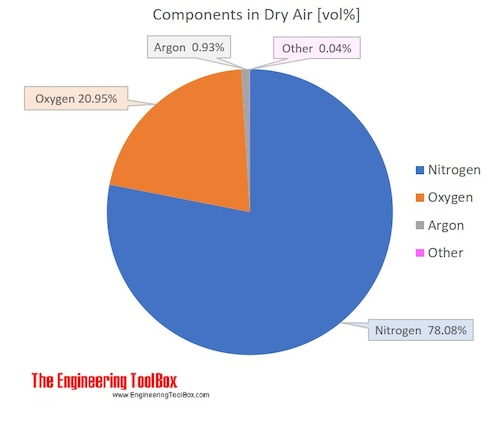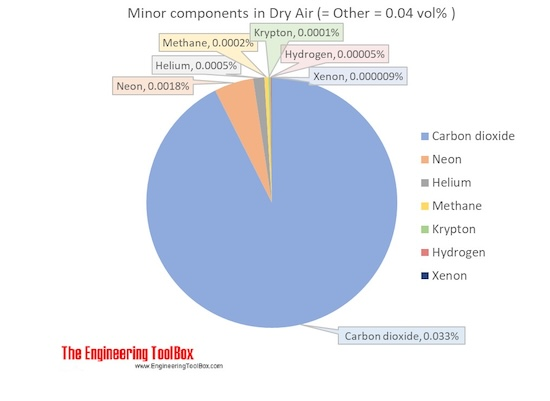Engineering ToolBox - Resources, Tools and Basic Information for Engineering and Design of Technical Applications!

# Air - Molecular Weight and Composition

## Dry air is a mixture of gases where the average molecular weight (or molar mass) can be calculated by adding the weight of each component.

The molecular weight (or molar mass) of a substance is the mass of one mole of the substance, and can be calculated by summarizing the molar masses of all the atoms in the molecule.

### Components in Dry Air

Air is a mixture of several gases, where the two most dominant components in dry air are 21 vol% oxygen and 78 vol% nitrogen. Oxygen has a molar mass of 15.9994 g/mol and nitrogen has a molar mass of 14.0067 g/mol. Since both of these elements are diatomic in air - O2 and N2, the molar mass of oxygen gas is aprox. 32 g/mol and the molar mass of nitrogen gas is aprox. 28 g/mol.

The average molar mass is equal to the sum of the mole fractions of each gas multiplied by the molar mass of that particular gas:

Mmixture = (x1*M1 + ......+ xn*Mn)                                        (1)

where

xi = mole fractions of each gas
Mi = the molar mass of each gas

The molar mass of dry air with oxygen, nitrogen and the other components as indicated below is 28.9647 g/mol.  Composition and content of each gas in air is given in the figures and the table below.For full table with Molar mass an Molar mass in Air - rotate the screen!

 Components in dry air Volume ratio = Molar ratio,  compared to dry air Molar Mass Molar mass in air Name Formula [mol/molair] [vol %] [g/mol][kg/kmol] [g/molair][kg/kmolair] [wt %] Nitrogen N2 0.78084 78.084 28.013 21.873983 75.52 Oxygen O2 0.20946 20.946 31.999 6.702469 23.14 Argon Ar 0.00934 0.934 39.948 0.373114 1.29 Carbon dioxide CO2 0.00033 0.033 44.010 0.014677 0.051 Neon Ne 0.00001818 0.001818 20.180 0.000367 0.0013 Helium He 0.00000524 0.000524 4.003 0.000021 0.00007 Methane CH4 0.00000179 0.000179 16.042 0.000029 0.00010 Krypton Kr 0.0000010 0.0001 83.798 0.000084 0.00029 Hydrogen H2 0.0000005 0.00005 2.016 0.000001 0.000003 Xenon Xe 0.00000009 0.000009 131.293 0.000012 0.00004 Average molar mass of air 28.9647
• 1 kg = 2.2046 lb

### Air Density

The density of dry air can be calculated with the Ideal Gas Law

ρ = P / (R T)                              (1)

where

P = pressure (Pa)

Rair = 287.05 = individual gas constant [J/kg K]

T = absolute temperature [K]

Example: The density of dry air at atmospheric pressure 101.325 kPa (101325 Pa) and 0 oC (= 273.15 K) can be calculated as

ρ = 101325 [Pa] / (287.05 [J/kg K]*273.15 [K])

= 1.292 [kg/m3]

### Water Vapor

Water vapor is almost always present in air. The content may vary and the maximum amount possible of water vapor in dry air depends on the temperature of the air.

Water vapor - H2O - is composed of one oxygen atom and two hydrogen atoms. Hydrogen is the lightest element with a molar mass of 1 g/mol,  while oxygen has 16 g/mol. Thus, the water vapor atom has an molar mass of 18 g/mol, and water vapor is lighter than O2 with 32 g/mol and N2 with 28 g/mol.

• Note! Water vapor in air will dilute the other gases and reduce the total density of the mixture. Dry air is more dense than humid air!

The vapor in air may saturate to droplets when temperature is decreased or pressure is increased.

Humid air containing water molecules as liquid - droplets - may be more dense than dry air or humid air containing water only as vapor.

## Related Topics

• Air Psychrometrics - Moist and humid air - psychrometric charts, Mollier diagrams, air-condition temperatures and absolute and relative humidity and moisture content.
• Material Properties - Material properties of gases, fluids and solids - densities, specific heats, viscosities and more.
• Fluid Mechanics - The study of fluids - liquids and gases. Involving velocity, pressure, density and temperature as functions of space and time.
• Gases and Compressed Air - Air, LNG, LPG and other common gas properties, pipeline capacities, sizing of relief valves.

## Engineering ToolBox - SketchUp Extension - Online 3D modeling!

Add standard and customized parametric components - like flange beams, lumbers, piping, stairs and more - to your Sketchup model with the Engineering ToolBox - SketchUp Extension - enabled for use with the amazing, fun and free SketchUp Make and SketchUp Pro .Add the Engineering ToolBox extension to your SketchUp from the SketchUp Pro Sketchup Extension Warehouse!

Translate

## Privacy

We don't collect information from our users. Only emails and answers are saved in our archive. Cookies are only used in the browser to improve user experience.

Some of our calculators and applications let you save application data to your local computer. These applications will - due to browser restrictions - send data between your browser and our server. We don't save this data.

## Citation

• Engineering ToolBox, (2004). Air - Molecular Weight and Composition. [online] Available at: https://www.engineeringtoolbox.com/molecular-mass-air-d_679.html [Accessed Day Mo. Year].

Modify access date.

. .

#### Scientific Online Calculator3 10